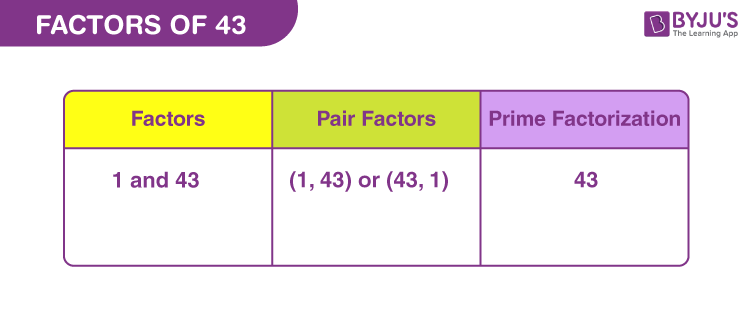# Factors of 43

Factors of 43 are the natural numbers that uniformly divide the original number. A factor is any number that divides another number evenly. The factors are also called divisors of a number. 43 is a prime number, therefore, it is divisible by 1 and the number itself.

Alternatively, we can say, if the multiplication of two positive natural numbers is equal to 43, then they are the factors of 43.## How to Find the Factors of 43?

To find the factors of a number, we need to divide the original number by the possible natural numbers that can produce zero remainder.

 43 ÷ 1 = 43 [Remainder = 0] 43 ÷ 43 = 1 [Remainder = 0]

Therefore, there are only two factors of 43. They are 1 and 43.

Let us check dividing 43 by any other number. If the resultant is not a whole number, then the divisor is not a fraction.

43 ÷ 2 = 21.5

43 ÷ 5 = 8.6

43 ÷ 15 = 2.87

Thus, there are no other factors for 43.

## Pair Factors of 43

Pair factors of 43 are the pair of numbers that produces the original number on multiplication.

• 1 × 43 = 43
• 43 × 1 = 43

Therefore, the pair factors of 43 are (1, 43) or (43, 1).

If we consider the negative factors in pairs to get 43, then;

• -1 × -43 = 43
• -43 × -1 = 43

Therefore, the negative pair factors are (-1, -43) or (-43, -1).

## Prime Factorisation of 43

43 is itself a prime number, therefore, it is only divisible by 43.

43 ÷ 43 = 1

Therefore, the prime factor of 43 is 43.

 Prime factorisation of 43 = 43 Exponential form = 431

## Video Lesson on Prime Factors## Solved Examples

Q.1: What is the sum of all the factors of 43?

Solution: The factors of 43 are 1 and 43.

Sum = 1 + 43 = 44

Therefore, the required sum is 44

Q.2: What are the common factors of 43 and 53?

Solution: We have to list the factors of 43 and 53.

43 → 1, 43

53 → 1, 53

Therefore, the common factor of 43 and 53 is 1.

Q.3: What are the greatest common factors of 43 and 129?

Solution: The factors of the respective numbers are:

43 → 1, 43

129 → 1, 3, 43, 129

Therefore, GCF of 43 and 129 is 43.

### Practice Questions

1. Find the GCF of 43 and 79.
2. What is the next prime number after 43?
3. What are the common factors of 43 and 44?
4. What is the similarity between 43 and 97?

## Frequently Asked Questions on Factors of 43

### What are the factors of 43?

The factors of 43 are 1 and 43. Hence, there are two factors.

### Is 43 a prime number?

Yes, 43 is a prime number, since it has only two factors.

### What are the multiples of 43?

The first ten multiples of 43 are 43, 86, 129, 172, 215, 258, 301, 344, 387 and 430.

### Can 43 be divided evenly?

43 does not have any other factors apart from 1 and itself, to divide it evenly.

### Is 13 a factor of 43?

No, 13 is not a factor 43 because 13 cannot divide 43, uniformly.